Plasma Accelerators

Plasma Accelerators

devices for producing plasma fluxes with velocities of 10—103 km/sec or more, corresponding to an ion kinetic energy of between ~ 10 and 10s—106 electron volts (eV). At the lower energy limit plasma accelerators border on low-temperature plasma generators, or plasmatrons; at the upper limit they border on collective charged-particle accelerators. Plasma accelerators are generally accelerators of completely ionized plasmas. Excitation, ionization, and thermal processes therefore play an ancillary role, in contrast to plasmatrons.

High-velocity plasma fluxes can be obtained by various methods, for example, by irradiating a solid body with a laser beam. Plasma accelerators in the strict sense, however, include only devices (Figure 1) in which the acceleration and usually the simultaneous production of the plasma are accomplished through electrical energy by means of one or several special electric discharges.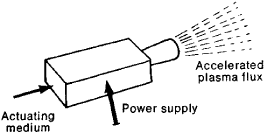Figure 1. Schematic of a plasma accelerator

In contrast to charged-particle accelerators, the channel of a plasma accelerator simultaneously contains particles with charges of both signs—positive ions and electrons; that is, violation of quasi-neutrality does not occur. This removes the limitations associated with a space charge and makes it possible to obtain plasma fluxes with an effective ion current of several million amperes (A) with a particle energy of ~ 100 eV. At ion fluxes of ~ 1,000 A, a particle energy of several thousand electron volts has already been achieved.

Ions and electrons emerge from plasma accelerators with practically equal directed velocities. Consequently, the ions—because of their large mass—have most of the energy of the flux. Plasma accelerators are thus electrical systems that accelerate ions in the presence of electrons that compensate for the space charge of the ions.

Acceleration mechanism. In analyzing the working process in a plasma accelerator, a plasma may be considered either as a continuous medium or as an ensemble of particles (ions and electrons). Within the framework of the first approach, the plasma acceleration is due to two factors: (1) a drop in the total pressure p = p1 + pe, where p1 and pe are the ion and electron pressures; and (2) the action of the electromagnetic force F, which arises from the interaction of the currents flowing in a plasma with a magnetic field—F ~ [jB], where j is the current density in the plasma and B is the magnetic field density.

Within the framework of the second approach, ion acceleration can occur as a result of (1) the action of the electric field E that exists in the plasma volume; (2) collisions of the directed flux of electrons with ions (the electron wind); (3) collisions of ions with ions, as a result of which energy of the random motion of ions becomes directed (thermal or gas-dynamic acceleration of the ions). The electrical acceleration of ions has the greatest importance for plasma accelerators; the second and third mechanisms are of less importance.

Classification of plasma accelerators. Plasma accelerators are divided into thermal and electromagnetic types, depending on whether the total pressure drop or the electromagnetic force predominates in the acceleration process.

The most important thermal plasma accelerators are noniso-thermal accelerators, in which pe≫ p1, because it usually is difficult to create a plasma with a high ion temperature T, and comparatively simple to create one with “hot” electrons (Te≫ T1). Such a plasma is nonisothermal. In design a nonisothermal accelerator is a “magnetic nozzle” (Figure 2) in which a plasma with hot electrons (T, ~ 107-109 °K or, in energy units, kTe~ 103—10s eV, where k is the Boltzmann constant) is created either by injecting fast electrons or by electron cyclotron resonance. In striving to leave the chamber, the electrons create an electric field of space charges that “extracts” (accelerates) ions; the field imparts to the ions an energy of the order of kTe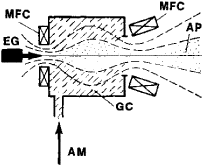Figure 2. Schematic of a nonisothermal plasma accelerator. The electron beam leaving the electron gun (EG) heats the electrons in the gas-discharge chamber (GC) and ionizes the actuating medium (AM) that is being fed into the chamber. The accelerated plasma (AP) resulting from the electron pressure drop flows out along the lines of magnetic force created by the magnetic field coils (MFC).

Electromagnetic plasma accelerators are subdivided according to the nature of the energy supply to the plasma. Three classes are distinguished: (1) radiation accelerators, in which acceleration of the plasma flux occurs as a result of the pressure of an electromagnetic wave incident on a plasma bunch (Figure 3,a); (2) induction accelerators, or pulsed systems in which a growing external magnetic field B induces a current j in a plasma ring (Figure 3,b) that can be created in various ways—the interaction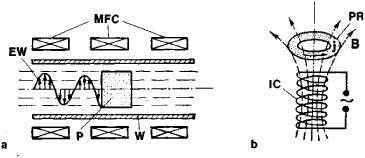Figure 3. (a) Schematic of a radiation plasma accelerator: (MFC) magnetic field coils, (W) wave guide, (P) plasma bunch, (EW) electromagnetic wave, (b) Schematic of an induction plasma accelerator: (B) magnetic field, (PR) plasma ring, (IC) induction coil, (j) current in plasma ring.

of this current with the radial component of the external magnetic field creates an electromagnetic force, which accelerates the plasma ring; (3) electrode plasma accelerators, in which there is direct contact between the plasma being accelerated and electrodes connected to the voltage source. Acceleration of the plasma occurs during the electromagnetic interaction of this current with an external magnetic field—that is, a field created by a separate magnetic system—or with the self-magnetic field created by the current flowing through the plasma. Electrode plasma accelerators are the best studied and most numerous type and will be considered in greater detail below.

Plasma accelerators with a self-magnetic field, PULSED ELECTRODE ACCELERATORS (GUNS). The first plasma accelerator was the rail-type accelerator (Figure 4,a), powered by a capacitor bank.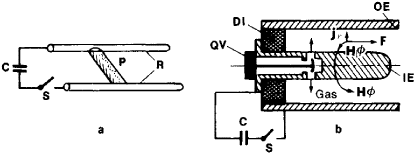Figure 4. (a) Schematic of a rail-type accelerator: (R) rails, (P) plasma bridge, (C) capacitor, (S) switch, (b) Schematic of a coaxial pulsed plasma accelerator. The quick-acting valve (QV) feeds gas to the gap between the inner electrode (IE) and the outer electrode (OE); (DI) dielectric insert between the electrodes. After the switch (S) is closed, a current that ionizes the gas arises in the circuit.

A plasma bunch is created by passing a large current through a thin wire stretched between rails, which thus is vaporized and ionized, or by ionizing gas fed into the interelectrode gap through a special valve. During the discharge the self-magnetic field of the electric circuit acts on the plasma-bridge current, which reaches tens or hundreds of thousands of amperes; as a result, acceleration of the bunch occurs in a time of the order of 1 microsecond. The form of a coaxial system later was given to pulsed accelerators (Figure 4,b). In this case the plasma bunch is accelerated under the action of the electromagnetic force F that arises during the interaction of the radial current component jr with the azimuthal self-magnetic field Hϕ. Such plasma accelerators have already found extensive application. They permit the obtaining of bunches with velocities of up to 108 cm/sec and with a total number of particles that reaches 1018.

STEADY-STATE STRONG-CURRENT END-TYPE ACCELERATORS. In principle a coaxial accelerator can be made steady-state (operating in continuous mode) if the actuating medium (the gas being ionized) is fed continuously into the gap between the electrodes. Because of the Hall effect, however, during a steady-state discharge in an accelerator the electric current has a significant longitudinal component. As a result, several effects occur; for example, the plasma is “squeezed out” toward the cathode and potential discontinuities are formed in the region of the anode. These effects lead to a sharp reduction in efficiency. The “end-type” scheme (Figure 5,a), wherein the actuating medium is fed simultaneously through a short cathode, is consequently more efficient. In an end-type plasma accelerator the plasma is also accelerated primarily by the electromagnetic force arising during the interaction of the radial component jr of the current j with the azimuthal magnetic field Hϕ. If with a constant supply of working substance the discharge current Ij is continuously increased, then the rate of plasma efflux and the efficiency of the accelerator will initially increase. At some value of /¿, however, a large part of the discharge current is carried beyond the end face of the accelerator, the voltage increases sharply, the efficiency decreases, and oscillations arise in the accelerator. A critical regime sets in. The pinch effect, as a result of which the plasma column is separated from the anode, is apparently the physical cause of the critical regime.

In normally operating end-type accelerators with a self-magnetic field, it is possible to produce, for a discharge current of about 104 A, steady-state plasma fluxes with velocities of ~ 100 km/sec and with characteristic flow rates of the actuating medium of —0.01–0.1 g/sec. Here the discharge voltage is about 50 volts.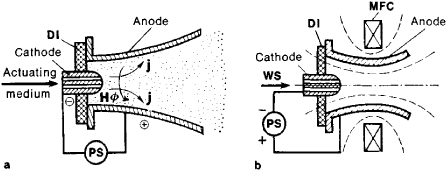Figure 5. (a) Schematic of an end-type plasma accelerator, (b) schematic of an end-type magnetic plasma accelerator; (DI) dielectric insert, (MFC) magnetic field coil, (PS) direct-current power supply, (AM) actuating medium

The end-type accelerator described above is inoperative not only at high but also at low discharge currents Id, since the electromagnetic force is proportional to Id2. Therefore, when Id < 1,000 A, the role of the electromagnetic force under real conditions becomes smaller than that of the gas kinetic pressure, and the end-type plasma accelerator is converted to a conventional plasmatron. In order to increase the efficiency of an end-type accelerator at low power levels, an external magnetic field is created in the working channel (Figure 5,b). The resultant accelerator is called an end-face Hall accelerator, or a magnetic plasma accelerator. It permits the generation of plasma fluxes with velocities of tens of km/sec given a power ≥ 10 kilowatts (kW). A noteworthy characteristic of end-type accelerators is that they can produce particle fluxes with an energy several times greater than the applied potential difference. In the terms of particle dynamics, this may be attributed to the entrainment of ions as a result of collisions with the electron flux from the cathode (the electron wind).

Plasma accelerators with an external magnetic field. If it is required to produce low-power (≲, 10 kW) steady-state fluxes or particle fluxes with high velocities (;≳ 10 cm/sec), closed-drift plasma accelerators, one version of which is illustrated in Figure 6, are particularly convenient. Such an accelerator is an axially symmetric system with a radial magnetic field in a circular acceleration channel that contains the plasma. The operation of this plasma accelerator can be understood more easily by considering the dynamics of the electrons and ions.

If a potential difference is applied between the anode and the cathode, then the electrons will begin to drift—that is, to move on the average with a constant velocity—perpendicular to the electric field E and the magnetic field H and will describe curves approximating cycloids. The acceleration channel length L is selected so that the height of the electron cycloid he is much less than L (L ≫ he). In this case we say that the electrons are “magnetized.” By virtue of the large mass (M1) of an ion, the height of the ion cycloid h1 is M1/me times greater than he, where me is the mass of the electron. Therefore, if the channel length L is made much smaller than h1, the ions will be slightly deflected by the magnetic field and will be accelerated by the electric field along a virtually straight line. The energy acquired by the ions in such an accelerator is close to the product of the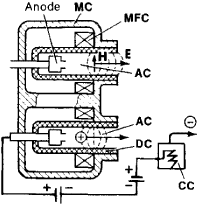Figure 6. Schematic of a plasma accelerator with closed drift. The magnetic field (H) is created by the magnetic circuit (MC) and the coils (MFC).

potential difference applied between the anode and cathode and the charge of the ion; the discharge current is close to the current of the accelerated ions.

Viewed as a whole, the action of a plasma accelerator of the type described is the following. The actuating medium in the form of a gas or vapor passes through the anode into the circular acceleration channel (AC, Figure 6). Here, a neutral atom is ionized after entering the cloud of electrons that are drifting azimuthally under the influence of the mutually perpendicular magnetic field H and electric field E. As a result of collisions with ions, atoms, and the wall of the dielectric chamber (DC, Figure 6) and under the action of the oscillations, the electron that arises in the process of ionization diffuses to the anode; the ion accelerated by the electric field leaves the channel. The space charge of the ions located in the channel is constantly counterbalanced by the charge of the drifting electrons. Consequently, here, in contrast to ion sources, there are no limitations on the magnitude of the ion current being “extracted.” Lest quasi-neu-trality be violated, an ion that has left the channel gains an electron from the compensator cathode (CC, Figure 6). There are a number of versions of plasma accelerators with closed drift —for example, accelerators with an anode layer and single- and multiple-lens accelerators. These accelerators permit the obtaining of plasma fluxes with an effective ion current ranging from a few units to many hundreds of amperes and with an energy of 102–104 eV or more.

Applications. The first plasma accelerators appeared in the mid-1950’s. Plasma accelerators have found application as plasma jet engines. They are used in the cathode sputtering technique for the cleaning of surfaces and in the coating of various surfaces with metal films. Other areas of application include investigations in ionospheric aerodynamics and experimental astrophysics (the modeling of space phenomena), thermonuclear research (as plasma injectors), and plasma chemistry. As the design is improved and new parameters are attained, the range of application of plasma accelerators will continually expand.

REFERENCE

Plazmennye uskoriteli. Edited by L. A. Artsimovich et al. Moscow, 1973.

A. I. MOROZOV

References in periodicals archive ?
To date most of experiments on laser plasma accelerators have been carried out by employing ultrashort pulse lasers with duration [t.
For applications of unique particle beam and radiation sources, one needs proper formulas for designing laser plasma accelerators to meet the requirement of electron beam parameters such as energy and charge, complying with the scaling law obtained from laser plasma accelerator experiments.
The state-of-the-art of PW-class lasers allows us to study the feasibility of laser plasma accelerators toward 100 GeV in a full scale experiment.
A design of the neutral gas-filled plasma waveguide for 100 GeV laser plasma accelerators has been presented in Ref.
100) More specifically, the achievements of research on laser plasma accelerators delivering electron beams having multi-GeV energy and high-qualities from a centimeter-scale plasma could inspire various applications in the broad range of sciences and technologies.
Tolok, a significant program on development of quasi-stationary plasma accelerators (QSPA) was started in KIPT, and the most powerful in the world plasma accelerator was put into operation in 1990, which is actively used in radiation technologies.
Mark Cappelli, a professor at Stanford University, presented, "Toward Reduced Wall-Effect Hall Plasma Accelerators.
The recent progress realized by laser plasma accelerators that can nowadays deliver high quality energetic particle beams in ultra short bunches (of a few femtoseds) with very high peak currents (of a few kA) are very encouraging for the future.
July 9-13 Laser and Plasma Accelerators Workshop 2007.
9 million program would support research in inertial fusion energy, fast ignition, petawatt laser development, plasma accelerators, and other research presently funded by Fusion Energy, Nuclear Physics, High Energy Physics, and inertial confinement fusion accounts within the National Nuclear Security Agency.
This office will be charged with supporting research in inertial fusion energy, fast ignition, petawatt laser development, plasma accelerators and other laboratory and university sponsored research related to high energy density science that is presently funded within the Fusion Energy, Nuclear Physics, High Energy Physics and the NNSA, ICF accounts.

Site: Follow: Share:
Open / Close